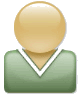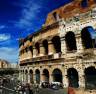TutorialAccountForums

## A Math Puzzle

Category: 99 Burning Lit

Level 1.

Each letter represents a digit - not a number.  So, for example, and example only (as you can see since there is no K in this puzzle), if K is 1 and Y is 0, then KY would be 10, not 1 * 0.
Also every two-digit number is greater than 9, and every three-digit number is greater than 99, and so on.  We don’t try to trick you by adding extra zeroes at the front of a number (or any other way, for that matter).

TU + TV = UN
O * P = YN
B * A = OA
TNN = TN * TN
I * P = OP

There is another place on the website that offers clues if you’re having difficulty, but for the pleasure of those who like to discover for themselves, I won’t tell you where unless you ask directly.

There are 11 puzzles at this time, and as interest picks up, I will release them.

There is a second-level puzzle you might like to solve, which will be very difficult.  If you’re interested in solving it, try to figure out how I decided on the letter-to-digit maps.  It’s the same strategy every time, on different parts of a very important quotation.  You’ll probably need more than the first puzzle to figure it out.  Just remember that a quotation reuses the same letters over and over again, so I couldn’t do a straight mapping, and I’ll explain later how to fix that.

When the puzzles are all solved, I’ll make an 11th one using the letters A - J, standing for the other puzzles.  The last step is to put the letters from each puzzle in order according to the digits they stand for.  You may notice words emerge.  Don’t be alarmed!  It was designed that way.  If you use the last puzzle to put the first ten in order (for example, in the 11th puzzle, if A represents a 3, then the 3rd puzzle goes first), then you’ll see the important quotation.

I might have made errors.  You get a free copy of the latest issue if you find one.

Posted by Dave Scotese on 06/04 at 05:05 PM | Permalink
(2) Discuss • (0) Comments